# HyperbolicAnisotropicEnumerateFunction¶

class HyperbolicAnisotropicEnumerateFunction(*args)

Hyperbolic and anisotropic enumerate function.

Available constructors:

HyperbolicAnisotropicEnumerateFunction(dim)

HyperbolicAnisotropicEnumerateFunction(dim, q)

HyperbolicAnisotropicEnumerateFunction(weight)

HyperbolicAnisotropicEnumerateFunction(weight, q)

Parameters:
diminteger

Dimension of the EnumerateFunction. dim must be equal to the dimension of the OrthogonalBasis.

qfloat

Correspond to the q-quasi norm parameter. If not precised,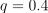.

weightsequence of float

Weights of the indices in each dimension. If not precised, all weights are equals to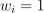.

Notes

The hyperbolic truncation strategy is inspired by the so-called sparsity-of- effects principle, which states that most models are principally governed by main effects and low-order interactions. Accordingly, one wishes to define an enumeration strategy which first selects those multi-indices related to main effects, i.e. with a reasonably small number of nonzero components, prior to selecting those associated with higher-order interactions.

For any real number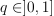, one defines the anisotropic hyperbolic norm of a multi-indexby: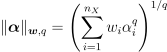where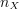is the number of input variables and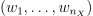is a sequence of real positive numbers called weights. Functions of input variables with smaller weights are selected first for the functional basis.

Examples

In the following example, we create an hyperbolic enumerate function in 2 dimension with a quasi-norm equal to 0.5. Notice, for example, that the function with multi-index [3,0] come before [1,1], although the sum of marginal indices is lower: this is the result of the hyperbolic quasi-norm.

>>> import openturns as ot
>>> enumerateFunction = ot.HyperbolicAnisotropicEnumerateFunction(2, 0.5)
>>> for i in range(10):
...     print(enumerateFunction(i))
[0,0]
[1,0]
[0,1]
[2,0]
[0,2]
[3,0]
[0,3]
[1,1]
[4,0]
[0,4]


In the following example, we create an hyperbolic enumerate function in 3 dimensions based on the weights [1,2,4]. Notice that the first marginal index, with weight equal to 1, comes first in the enumeration.

>>> import openturns as ot
>>> enumerateFunction = ot.HyperbolicAnisotropicEnumerateFunction([1, 2, 4])
>>> for i in range(20):
...     print('i=', i, 'enum=', enumerateFunction(i))
i= 0 enum= [0,0,0]
i= 1 enum= [1,0,0]
i= 2 enum= [0,1,0]
i= 3 enum= [2,0,0]
i= 4 enum= [3,0,0]
i= 5 enum= [0,0,1]
i= 6 enum= [0,2,0]
i= 7 enum= [4,0,0]
i= 8 enum= [5,0,0]
i= 9 enum= [0,3,0]
i= 10 enum= [6,0,0]
i= 11 enum= [7,0,0]
i= 12 enum= [0,0,2]
i= 13 enum= [0,4,0]
i= 14 enum= [8,0,0]
i= 15 enum= [1,1,0]
i= 16 enum= [9,0,0]
i= 17 enum= [0,5,0]
i= 18 enum= [10,0,0]
i= 19 enum= [11,0,0]


Methods

 __call__(index) Call self as a function. getBasisSizeFromTotalDegree(maximumDegree) Get the basis size corresponding to a total degree. Accessor to the object's name. Return the dimension of the EnumerateFunction. Accessor to the object's id. getMaximumDegreeCardinal(maximumDegree) Get the number of terms of total degree below a threshold. getMaximumDegreeStrataIndex(maximumDegree) Get the largest index of the strata containing terms of maximum degree. Accessor to the object's name. Accessor to the norm. Accessor to the object's shadowed id. getStrataCardinal(strataIndex) Get the number of terms in the basis inside a given strata. getStrataCumulatedCardinal(strataIndex) Get the number of terms in the basis inside a range of stratas. Accessor to the upper bound. Accessor to the object's visibility state. Accessor to the weights. Test if the object is named. Test if the object has a distinguishable name. inverse(indices) Get the antecedent of a indices list in the EnumerateFunction. setDimension(dimension) Set the dimension of the EnumerateFunction. setName(name) Accessor to the object's name. Accessor to the norm. Accessor to the object's shadowed id. setUpperBound(upperBound) Accessor to the upper bound. setVisibility(visible) Accessor to the object's visibility state. setWeight(weight) Accessor to the weights.
__init__(*args)
getBasisSizeFromTotalDegree(maximumDegree)

Get the basis size corresponding to a total degree.

Parameters:
max_degint

Maximum total degree.

Returns:
sizeint

Number of terms in the basis of total degree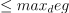.

Notes

In the specific context of a linear enumeration (LinearEnumerateFunction) this is also the cumulated cardinal of stratas up to max_deg.

Examples

>>> import openturns as ot
>>> dim = 2
>>> enum_func = ot.LinearEnumerateFunction(dim)
>>> enum_func.getBasisSizeFromTotalDegree(3)
10
>>> enum_func.getStrataCumulatedCardinal(3)
10

getClassName()

Accessor to the object’s name.

Returns:
class_namestr

The object class name (object.__class__.__name__).

getDimension()

Return the dimension of the EnumerateFunction.

Returns:
dimint,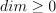Dimension of the EnumerateFunction.

getId()

Accessor to the object’s id.

Returns:
idint

Internal unique identifier.

getMaximumDegreeCardinal(maximumDegree)

Get the number of terms of total degree below a threshold.

Parameters:
max_degint

Maximum total degree.

Returns:
cardinalint

Number of terms in the basis of total degree.

Notes

In the specific context of a linear enumeration (LinearEnumerateFunction) this is also the cumulated cardinal of stratas of index.

Examples

>>> import openturns as ot
>>> dim = 2
>>> enum_func = ot.LinearEnumerateFunction(dim)
>>> enum_func.getMaximumDegreeCardinal(2)
6

getMaximumDegreeStrataIndex(maximumDegree)

Get the largest index of the strata containing terms of maximum degree.

Parameters:
max_degint

Maximum total degree.

Returns:
indexint

Index of the last strata that contains terms of total degree.

Notes

In the specific context of a linear enumeration (LinearEnumerateFunction) this is the strata of index max_deg.

Examples

>>> import openturns as ot
>>> dim = 2
>>> enum_func = ot.LinearEnumerateFunction(dim)
>>> enum_func.getMaximumDegreeStrataIndex(2)
2

getName()

Accessor to the object’s name.

Returns:
namestr

The name of the object.

getQ()

Accessor to the norm.

Returns:
qfloat

q-quasi norm parameter.

Accessor to the object’s shadowed id.

Returns:
idint

Internal unique identifier.

getStrataCardinal(strataIndex)

Get the number of terms in the basis inside a given strata.

Parameters:
strataIndexint

Index of the strata of the tensorized basis.

Returns:
cardinalint

Number of terms in the basis inside the strata strataIndex.

Notes

In the specific context of a linear enumeration (LinearEnumerateFunction) the strata strataIndex consists of a hyperplane of all the terms of total degree strataIndex, and its cardinal is strataIndex + 1.

Examples

>>> import openturns as ot
>>> dim = 2
>>> enum_func = ot.LinearEnumerateFunction(dim)
>>> enum_func.getStrataCardinal(2)
3

getStrataCumulatedCardinal(strataIndex)

Get the number of terms in the basis inside a range of stratas.

Parameters:
strataIndexint

Index of the strata of the tensorized basis.

Returns:
cardinalint

Number of terms in the basis inside the stratas of index lower of equal to strataIndex.

Notes

The number of terms is the total of terms inside each consecutive stratas. In the specific context of a linear enumeration (LinearEnumerateFunction) this returns the number of terms of maximal total degree strataIndex.

Examples

>>> import openturns as ot
>>> dim = 2
>>> enum_func = ot.LinearEnumerateFunction(dim)
>>> enum_func.getStrataCumulatedCardinal(2)
6
>>> sum([enum_func.getStrataCardinal(i) for i in range(3)])
6

getUpperBound()

Accessor to the upper bound.

Returns:
ubsequence of int

Upper bound of the indices (inclusive).

getVisibility()

Accessor to the object’s visibility state.

Returns:
visiblebool

Visibility flag.

getWeight()

Accessor to the weights.

Returns:
wPoint

Weights of the indices in each dimension.

hasName()

Test if the object is named.

Returns:
hasNamebool

True if the name is not empty.

hasVisibleName()

Test if the object has a distinguishable name.

Returns:
hasVisibleNamebool

True if the name is not empty and not the default one.

inverse(indices)

Get the antecedent of a indices list in the EnumerateFunction.

Parameters:
multiIndexsequence of int

List of indices.

Returns:
antecedentint

Represents the antecedent of the multiIndex in the EnumerateFunction.

Examples

>>> import openturns as ot
>>> dim = 2
>>> enum_func = ot.LinearEnumerateFunction(dim)
>>> for i in range(6):
...     print(str(i)+' '+str(enum_func(i)))
0 [0,0]
1 [1,0]
2 [0,1]
3 [2,0]
4 [1,1]
5 [0,2]
>>> print(enum_func.inverse([1,1]))
4

setDimension(dimension)

Set the dimension of the EnumerateFunction.

Parameters:
dimint,Dimension of the EnumerateFunction.

setName(name)

Accessor to the object’s name.

Parameters:
namestr

The name of the object.

setQ(q)

Accessor to the norm.

Parameters:
qfloat

q-quasi norm parameter.

Accessor to the object’s shadowed id.

Parameters:
idint

Internal unique identifier.

setUpperBound(upperBound)

Accessor to the upper bound.

Parameters:
ubsequence of int

Upper bound of the indices (inclusive).

setVisibility(visible)

Accessor to the object’s visibility state.

Parameters:
visiblebool

Visibility flag.

setWeight(weight)

Accessor to the weights.

Parameters:
wsequence of float

Weights of the indices in each dimension.

## Examples using the class¶